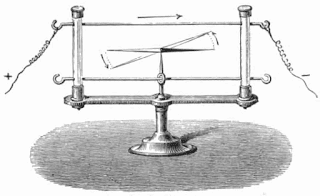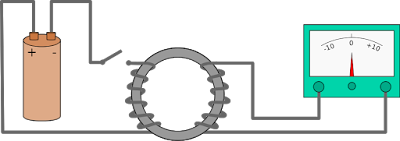# What Is Electro-magnetic Induction? Faraday Law Of Induction Explained !!Faraday’s electromagnetic induction experiment showing induction between coils of wire Credit: Wikimedia Commons

## What Is Electromagnetic Induction?

Electromagnetic (electricity and magnetism) induction is a phenomenon that produces an electromotive force (i.e, voltage) across an electric conductor when placed in the changing magnetic field. In simple terms, an emf is produced when a conductor is placed in a fluctuating magnetic field. Electromagnetic induction sometimes also called just an Induction or Faraday’s Law Of Induction.

## History Of Electromagnetic InductionØrsted’s experiment demonstrating that electric currents create magnetic fields Credit: Wikimedia Commons

Although Michael Faraday an English scientist, is generally credited for the discovery of electromagnetic induction, it was Hans Christian Ørsted a Danish physicist who was the first individual to discover that electric current creates magnetism, which is referred as the first connection found between electricity and magnetism. Soon after one year of Faraday’s discovery, an American scientist Joseph Henry too independently discovered electromagnetic induction phenomena.

## Discovery Of Electromagnetic Induction

On August 29, 1831; Michael Faraday discovered electromagnetic induction. In his experimental demonstration, he simply wrapped two wires on the iron ring (opposite sides as shown in the figure below). Faraday’s arrangement for the experiment was quite similar to the modern-day toroidal transformer. He expected that when he will connect the battery, the current will flow through the one wire (of an iron ring) and therefore, as a result, some kind of wave would travel across the ring producing some electrical effect on the opposite side of an iron ring. He also connected the other wire into a Galvanometer and watched it to calculate that what will happen next?A diagram of Faraday’s iron ring experiment. Change in the magnetic flux of the left side of the coil induces a current in the right side of the coil Credit: Wikimedia Commons

He saw a current flowing on two occasions: first– when he connected the wire to the battery and second– when he disconnected the wire from the battery and called the flowing current “wave of electricity“. He calculated that the current was induced due to the magnetic flux; occurred when the battery was connected and disconnected simultaneously (change in the magnetic field). The induction phenomenon discovered by Michael Faraday is also known as Faraday’s law of electromagnetic induction. Within the first two-three months of Faraday’s discovery, he found some other illustrations of electromagnetic induction such as Faraday’s disk.

Faraday also demonstrated that electromagnetic induction works reverse as well that is a moving electric charge can also generate magnetic field the same as the magnetic field generates an electric charge.

## Faraday’s Law Of Electromagnetic Induction

when the flux magnetic field passes through the area bounded by a closed conducting loop charges, an emf (electromagnetic force) is produced in the loop.

the emf is given bywhere,

ε = induced voltage in volts
N = no. of turns in the wire coil
t = time in second
andis the flux of the magnetic field through the area.

where
B = magnetic field
dA = area vector

When Faraday discovered his law of electromagnetic induction, it was widely criticized by most scientists and physicists at that time due to the inability of Faraday to formulate it mathematically. It was James Clerk Maxwell a Scottish scientist and mathematical physicist, who used Faraday’s ideas as the basis of his classical electromagnetic theory. Faraday’s collaboration with James Clerk Maxwell laid the foundation for the world-famous Maxwell’s equations.

Faraday’s Law Of Induction was mathematically described and generalized by James Clerk Maxwell into the maxwell-faraday equation; now listed as one of Maxwell’s equations.where,
∇× = curl operator
E = electric field ( a vector quantity)
B = magnetic field ( a vector quantity)

## Applications Of Faraday’s Law Of Induction

Faraday’s law of electromagnetic induction is a basic law of Electromagnetism. As it demonstrates how the magnetic field interacts with an electric current to produce (induced) electromagnetic force. There are so many listed application of Faraday’s law of electromagnetic induction such as
1. transformer
2. inductors
3. generators
4. electric motors
5. solenoid etc.